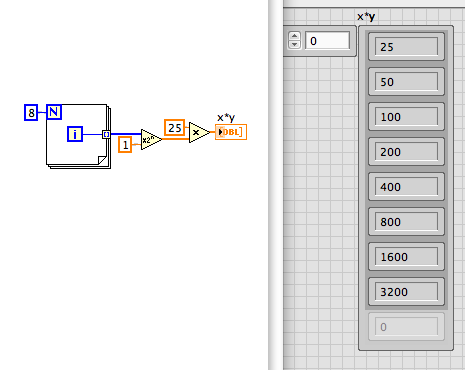# LabVIEW

cancel
Showing results for
Did you mean:

Solved!
Go to solution

## Formula Node Calculation Issues

I'm trying to make an array where the output is defined by the formula 25*(2^x) where x is the input variable, with integer iterations from 0 to 7.  To do this, I made an formula node and put it in a for loop.  Unfortunately the (^) symbol is not working right for the calculation.  I tested it simply with x^2 and this did not work, while having x*x returned the correct values.  Instead of outputting the correct values, it outputs seemingly random integers.  Anyone know why?  I am new to this software so I apologize if this this a common issue but I could not find help anywhere else.

Message 1 of 4
(942 Views)
Highlighted
Solution
Accepted by topic author DK08

## Re: Formula Node Calculation Issues

The LabVIEW Help does explain the allowed operators (Help>Formula Nodes>Precedence of Operators). The '^' is a bit exclusive or. A '**' is exponentiation.

Message 2 of 4
(938 Views)
Highlighted

## Re: Formula Node Calculation Issues

Thank you so much, I thought I was going crazy.

Message 3 of 4
(934 Views)
Highlighted

## Re: Formula Node Calculation Issues

If you use the numeric primitives rather than a formula node, craziness can be avoided (well, sometimes).

LynnMessage 4 of 4
(925 Views)